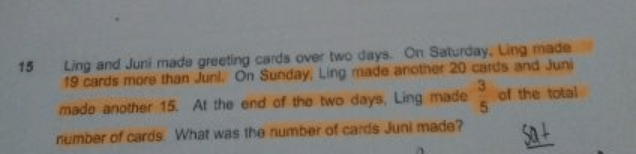# QuestionSaturday

Ling – 1 unit + 19

Juni – 1 unit

Sunday

Ling – 1 unit + 39 (3/5)

Juni – 1 unit + 15 (2/5)

Using Cross Multiplication,
(1 unit + 39) x 2 = (1 unit + 15) x 3

2 units + 78 = 3 units + 45

1 unit = 33

Juni = 33 + 15 = 48